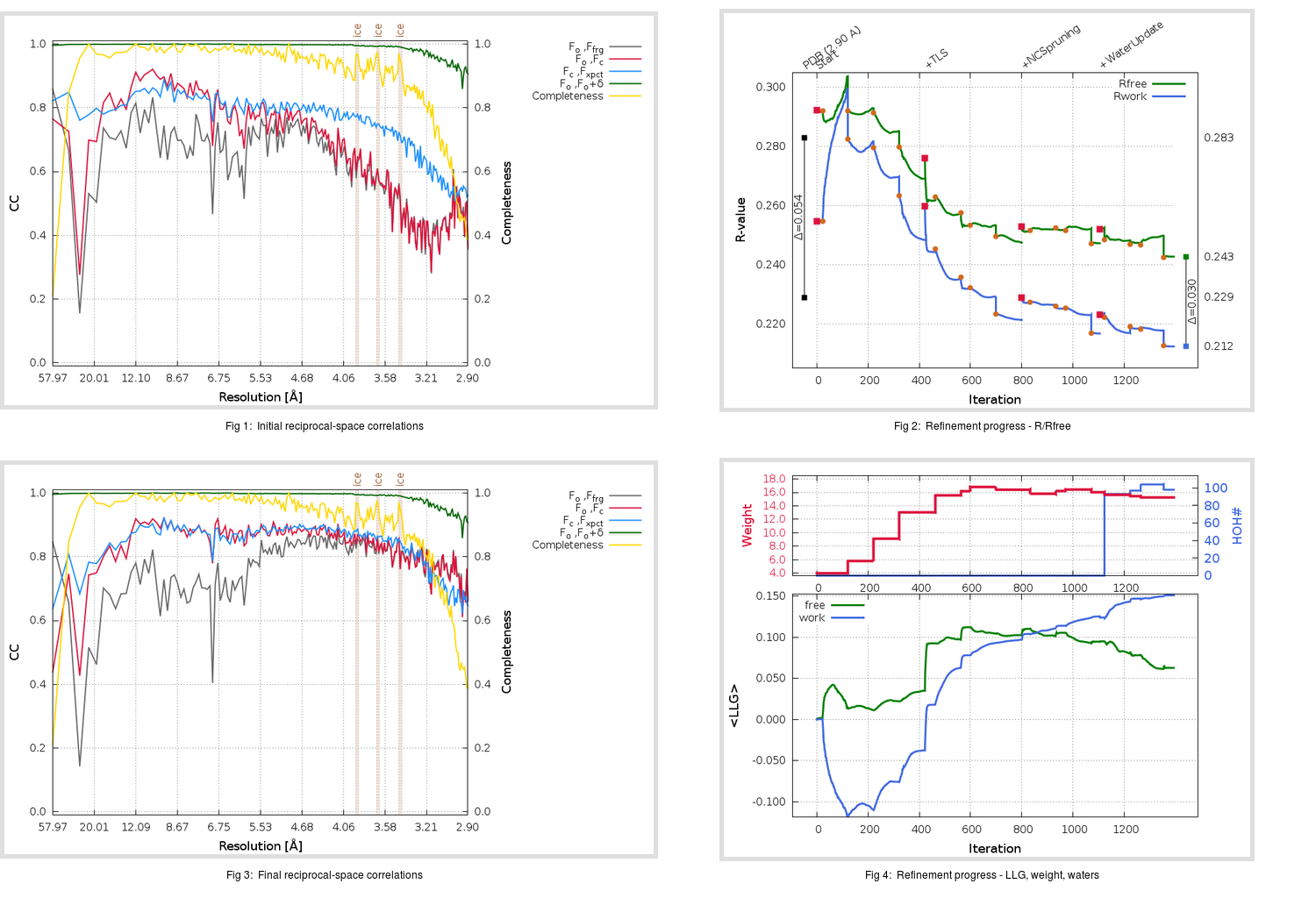Content:

```    Diffraction limits & principal axes of ellipsoid fitted to diffraction cut-off surface:
3.175         0.7338   0.0000   0.6793       0.641 a* + 0.768 c*
2.893         0.0000   1.0000   0.0000       b*
2.824        -0.6793   0.0000   0.7338      -0.540 a* + 0.841 c*
```

## Deposited

` `
 Date deposited Date data collection Resolution R, Rfree 20110607 2.90 0.2260 0.2830

Molprobity (CCP4 7.0 version) summary:

```Ramachandran outliers =   3.01 %
favored =  84.18 %
Rotamer outliers      =  12.00 %
C-beta deviations     =     5
Clashscore            =  14.78
RMS(bonds)            =   0.0099
RMS(angles)           =   1.27
MolProbity score      =   3.17
Resolution            =   2.90
R-work                =   0.2260
R-free                =   0.2830
```

```Number of waters      =     0

<B> (all atoms) =   79.46 ( sd =   22.93 ) for      12552 non-hydrogen atoms
<B>   (protein) =   79.46 ( sd =   22.93 ) for      12548 non-hydrogen atoms
<B>     (water) =    0.00 ( sd =    0.00 ) for          0 non-hydrogen atoms
<B>    (others) =   94.56 ( sd =    7.65 ) for          4 non-hydrogen atoms

B min/max       (all non-hydrogen atoms) =   34.51 /  139.16
B min/max   (protein non-hydrogen atoms) =   34.51 /  139.16
B min/max     (water non-hydrogen atoms) =    0.00 /    0.00
B min/max     (other non-hydrogen atoms) =   87.21 /  107.35
```

## BUSTER (re-)refinement

` `

Molprobity (CCP4 7.0 version) summary:

```Ramachandran outliers =   0.98 %
favored =  93.40 %
Rotamer outliers      =  14.29 %
C-beta deviations     =    16
Clashscore            =   8.41
RMS(bonds)            =   0.0121
RMS(angles)           =   1.58
MolProbity score      =   2.76
Resolution            =   2.90
R-work                =   0.2124
R-free                =   0.2428
```

```Number of waters      =    98

<B> (all atoms) =  115.30 ( sd =   32.33 ) for      12650 non-hydrogen atoms
<B>   (protein) =  115.59 ( sd =   32.27 ) for      12548 non-hydrogen atoms
<B>     (water) =   78.63 ( sd =   14.63 ) for         98 non-hydrogen atoms
<B>    (others) =   99.74 ( sd =    8.17 ) for          4 non-hydrogen atoms

B min/max       (all non-hydrogen atoms) =   46.83 /  244.24
B min/max   (protein non-hydrogen atoms) =   61.85 /  244.24
B min/max     (water non-hydrogen atoms) =   46.83 /  145.89
B min/max     (other non-hydrogen atoms) =   93.21 /  113.74
```

Refinement progression:Results:

` `
 File Remark 3SCI_aB_refine.01_04_refine.pdb.gz exact refinement commands are in header 3SCI_aB_refine.01_04_refine.mtz.gz including original deposited data and several re-refinement map coefficients 3SCI_aB_refine.01_04_BUSTER_model.cif.gz including any non-standard compound restraints 3SCI_aB_refine.01_04_BUSTER_refln.cif.gz